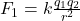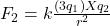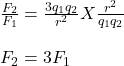## Two charges q1 and q2 are separated by a distance, d, and exert a force, F, on each other.Which best represents the new force F′ if q1 is tr

Question

Two charges q1 and q2 are separated by a distance, d, and exert a force, F, on each other.Which best represents the new force F′ if q1 is tripled?

in progress 0
6 months 2021-08-05T01:14:53+00:00 2 Answers 10 views 0

## Answers ( )

F
′ = 3 F

Explanation:

2. If the charge q₁ is tripled  then the force between the charged becomes 3 times the original charge.

Explanation:

Given:

Distance between the two charges = r

The force between two charges is given by:If q₁ is tripled, then the force F becomes:Therefore, if the charge q₁ is tripled  then the force between the charged becomes 3 times the original charge.